A Source Book in Classical Analysis

Contents:
Author: E. Picard  | Date: 1890

Mathematics

# Picard on the Picard Method

1

Let us take a single first-order equation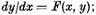then, setting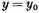when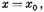one can establish the fundamental existence theorem for this equation. To this end, consider the equations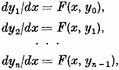effecting each quadrature2 in such a way that for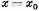one has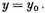The problem is to prove that, as n → ∞, yn tends to a limit y which represents the desired integral provided that x remains in the neighborhood of x0. We assume that the function F(x, y) is continuous and defined [on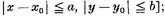moreover, that one can determine a positive constant k such that3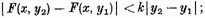we also assume that the function and the variables are real.

Let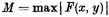when x and y remain between the indicated limits. One will have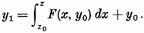Let ρ be a quantity nearly equal to a; y1 will stay within the desired limits if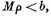and it is evident that the same will be true for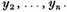Letting δ denote a quantity nearly equal to ρ, we will suppose that . . .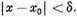251

Putting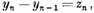we then have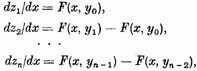and all the z vanish at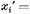One has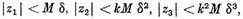and generally,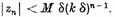Hence, writing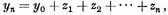one sees that yn tends to a limit if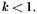As a decreasing geometric progression, the series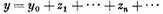will be convergent. Thus yn converges to a limit y when . . .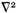δ being the smallest of the quantities a, b/M, 1/k.

In this interval, y evidently represents a continuous function of x. Thus one has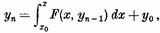and, as yn and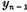tend to y, it follows that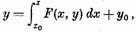and hence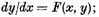that is, the limit y satisfies the differential equation. Thus, the existence of the solution has been established. One can evidently employ the same type of proof if F is an analytic function of the complex variables z and w.

1 E. Picard, "Mémoire sur la théorie des équations aux derivées partielles et la méthode des approximations successives," J. math. pures appl. 6 (1890), 145–210 [198–200].

2 What is meant is, choosing each constant of integration.

3 Picard here uses the Lipschitz condition of Selection 48.

Contents:

### Related Resources

None available for this document.

Title: A Source Book in Classical Analysis

Select an option:

*Note: A download may not start for up to 60 seconds.

## Email Options

Title: A Source Book in Classical Analysis

Select an option: